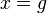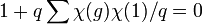# Conjugacy class of prime power size implies not simple

## Statement

If a finite group contains a Conjugacy class (?) whose size is a power of a prime (and also, greater than 1), then the finite group cannot be simple.

## Applications

This is used to prove that order has only two prime factors implies solvable -- the result commonly called Burnside's$p^aq^b$-theorem.

## Facts used

The table below lists key facts used directly and explicitly in the proof. Fact numbers as used in the table may be referenced in the proof. This table need not list facts used indirectly, i.e., facts that are used to prove these facts, and it need not list facts used implicitly through assumptions embedded in the choice of terminology and language.
Fact no. Statement Steps in the proof where it is used Qualitative description of how it is used What does it rely on? Difficulty level Other applications
1 Column orthogonality theorem which states that any two distinct columns in the character table of a finite group are orthogonal. Step (1) Provides the basic equation setup whose terms we then deduce more facts about. Basic linear representation theory click here
2 Zero-or-scalar lemma (which follows from the fact that size-degree-weighted characters are algebraic integers): If the size of the conjugacy class of$g \in G$ and the degree of an irreducible representation$\varphi$ with character$\chi$ are relatively prime, then either$\varphi(g)$ is a scalar matrix (where$\varphi$ is the linear representation associated to$\chi$) or$\chi(g) = 0$. Step (6) Shows that many terms are either zero or divisible by the prime$q$. Algebraic number theory + linear representation theory 4 click here
3 Characters are algebraic integers Step (9) Build-up to contradiction from assumption that$G$ is simple. Basic linear representation theory 2 click here

## Proof

This proof uses a tabular format for presentation. Provide feedback on tabular proof formats in a survey (opens in new window/tab) | Learn more about tabular proof formats|View all pages on facts with proofs in tabular format

Given: A finite group$G$, a prime number$q$, and a conjugacy class$C$ in$G$ of size$q^d$ where$q$ is a prime number and$d > 0$.$g \in C$ is a representative element.

To prove:$G$ is not a simple group.

Proof: Note that when the symbol$1$ appears as an input to a representation or a character, it refers to the identity element of$G$. When it appears as the output of a character, or in another context, it refers to the real number$1$.

Step no. Assertion/construction Facts used Given data used Previous steps used Explanation Commentary
1 We have$\! \sum_\chi \chi(g)\chi(1) = 0$ where the summation is over all irreducible characters of$G$ over the complex numbers. Note that this summation includes the trivial character, for which the corresponding summand is$1$. Fact (1)$C$ is a nontrivial conjugacy class (since its size is$q^d, d > 0$, it cannot be the trivial conjugacy class) [SHOW MORE] Use column orthogonality to get the equation with which we'll play havoc.
2 For any faithful linear representation of$G$ and any$x \in G$ such that$\varphi(x)$ is scalar,$x$ must be in the center of$G$. [SHOW MORE] Building toward step (5)
3 If$G$ is simple, then it is centerless.$G$ is non-abelian (on account of having a conjugacy class of size greater than 1) [SHOW MORE] Building toward step (5)
4 If$G$ is simple, then any nontrivial linear representation of$G$ is faithful. [SHOW MORE] Building toward step (5)
5 If$G$ is simple, then, for any nontrivial linear representation$\varphi$ of$G$,$\varphi(g)$ is not a scalar matrix$g \in C \subseteq G$$C$ is a nontrivial conjugacy class
Steps (2), (3), (4) We combine the steps, setting$x = g$ in Step (2). Obtain that the image of a particular element under a nontrivial linear representation is not a scalar.
6 Suppose$\varphi$ is a nontrivial irreducible linear representation of$G$ over$\mathbb{C}$ whose degree is not a multiple of$q$, with character$\chi$, then either$\chi(g) = 0$ or$\varphi(g)$ is a scalar. Fact (2) The conjugacy class$C$ of$g$ in$G$ has size a power of$q$ [SHOW MORE] Combine the prime power size of conjugacy class with the zero-or-scalar lemma. (Note that nontriviality is not essential here, but it is essential for combining with Step (5)).
7 If$G$ is simple, then for every nontrivial irreducible character$\chi$ of$G$, either$q$ divides$\chi(1)$ or$\chi(g) = 0$. Steps (5), (6) [SHOW MORE] Force character values for$q'$-degrees to be zero.
8 If$G$ is simple, we get the following:$\! 1 + q\sum \chi(g)\chi(1)/q = 0$ with the sum taken over all nontrivial irreducible characters$\chi$ of$G$ over$\mathbb{C}$ for which$q|\chi(1)$. Steps (1), (7) [SHOW MORE] Bring back the column orthogonality relation from Step (1)
9 Let$\alpha$ be the sum$\sum \chi(g)\chi(1)/q$, the sum being taken over all nontrivial irreducible characters$\chi$ of$G$ over$\mathbb{C}$ for which$q|\chi(1)$. Then,$\alpha$ is an algebraic integer. Fact (3) [SHOW MORE] Now it is an algebraic integer ...
10 If$G$ is simple,$\alpha = -1/q$ Steps (8), (9) [SHOW MORE] ... and now it isn't.
11 If$G$ is simple, we have a contradiction. Hence,$G$ is not simple. Steps (9), (10) [SHOW MORE] contradiction clincher.